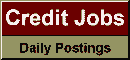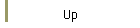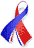DefaultRisk.com the web's biggest credit risk modeling resource.doi> search: A or B Export citation to:- HTML- Text (plain)- BibTeX- RIS- ReDIF

Correlation: Pitfalls and Alternatives

by Paul Embrechts of the ETH Zentrum,
Alexander McNeil of the ETH Zentrum, and
Daniel Straumann of the ETH Zentrum

March 1999

Introduction: Correlation is a minefield for the unwary. One does not have to search far in the literature of financial risk management to find misunderstanding and confusion. This is worrying since correlation is a central technical idea in finance.

Correlation lies at the heart of the capital asset pricing model (CAPM) and the arbitrage pricing theory (APT), where its use as a measure of dependence between financial instruments is essentially founded on an assumption of multivariate normally distributed returns. Increasingly, however, correlation is being used as a dependence measure in general risk management, often in areas where the assumption of multivariate normal risks is completely untenable - such as credit risk. In using correlation as an all-purpose dependence measure and transferring CAPM thinking to general risk management, many integrated risk management systems are being built on shaky foundations.

This article will tell you when it is safe and unproblematic to use correlation in the way that you imagine you can use it, and when you should take care. In particular it will tell you about two fallacies that have claimed many victims. These traps are known to statisticians, but not, we suggest, to the general correlation-using public. We will help you avoid these pitfalls and introduce an alternative approach to understanding and modelling dependency - copulas.

The recognition that correlation is often an satisfactory measure of dependence in financial risk management is not in itself new. For instance, Blyth (1996) and Shaw (1997) have made the point that (linear) correlation cannot capture the non-linear dependence relationships that exist between many real world risk factors. We believe this point is worth repeating and amplifying; our aim is to provide theoretical clarification of some of the important and often subtle issues surrounding correlation.

To keep things simple we consider only the static case. That is, we consider a vector of dependent risks (X1; : : : ;Xn)' at a fixed point in time. We do not consider serial correlation within or cross correlation between stochastic processes (see Boyer, Gibson, and Loretan (1999) in this context). Nor do we consider the statistical estimation of correlation, which is fraught with difficulty. In this paper we do not go this far because enough can go wrong in the static case to fill an entire book.

We require only simple mathematical notation. A risk Xi has marginal distribution Fi(x) = P {Xi <= x}. A vector of risks has joint distribution function F(x1, ... , xn) = P {X1 <= x1, ... , Xn <= xn}. The linear correlation of two risks is rho(Xi, Xj). For full mathematical details of the ideas we describe consult Embrechts, McNeil, and Straumann (1999).

Published in: RISK, Vol. 12, No. 5, (May 1999), pp. 69-71.

Books Referenced in this paper:  (what is this?)